# Exercise 11.2 Mensuration- NCERT Solutions Class 8

## Chapter 11 Ex.11.2 Question 1

The shape of the top surface of a table is a trapezium. Find its area if its parallel sides are $$1\,\rm{ m}$$ and $$1.2\,\rm{ m}$$ and perpendicular distance between them is $$0.8\rm{ m}.$$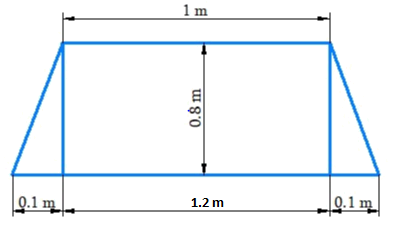### Solution

What is Known?

Shape of the table is trapezium and dimensions of the table.

What is unknown?

Area of the table.

Reasoning:

The table is rectangular in the middle and triangle at the end. Usually the area of the problem is sum of areas of two right angle triangle and rectangle.

Steps:

Base of the triangles

\begin{align}(CE+FD) &= (CD-EF)\\&= 1.2\;\rm{cm} – 1\,\rm{m} \\&= 0.2\,\rm{m}\end{align}

Base of one triangle \begin{align} = {\rm{CE}} = {\rm{FD}} = \frac{{0.2}}{2} = 0.1{\rm{m}} \end{align}

Height of the triangle $$= AE = BF = 0.3\,\rm{m}$$

Area of the triangle $$ACE$$ $$=$$ Area of the triangle $$BDF$$
\begin{align} &= \frac{1}{2} \times \rm{base} \times \rm{height}\\& = \frac{1}{2} \times 0.1{\rm{m}} \times 0.8{\rm{m}} = 0.04{{\rm{m}}^2}\end{align}

Area of the rectangle $$ABEF$$
\begin{align} &= 1{\rm{m}} \times 0.8{\rm{m}}\\ &= 0.8\,\rm{m^2}\end{align}

Therefore,
Area of the table $$=$$ Area of the triangle $$ACE$$ $$+$$ Area of the rectangle $$ABEF$$ $$+$$ Area of the triangle $$BFD$$

\begin{align}&= 0.04\,{{\rm{m}}^2} \times 0.8\,{{\rm{m}}^2} + 0.04\,{{\rm{m}}^2}\\&= 0.88\,{{\rm{m}}^2}\end{align}

## Chapter 11 Ex.11.2 Question 2

The area of a trapezium is$$\,{\rm{34}}\,\,{\rm{c}}{{\rm{m}}^2}$$ and the length of one of the parallel sides is $$10\, \rm{cm}$$ and its height is $$4 \,\rm{cm.}$$ Find the length of the other parallel side.

### Solution

What is Known?

Area, length of the one of the parallel sides of the trapezium and its height.

What is unknown?

Length of the other parallel side

Reasoning:

Since area of the trapezium is known. So is easy to find another parallel side by using given information.

Steps:

Area of the trapezium $$ABCD$$

$$= \frac{1}{2} \times$$(Sum of parallel side) $$\times$$Distance between its parallel sides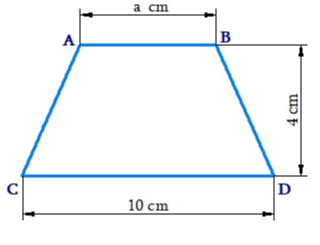\begin{align}34 &= \frac{1}{2} \times (AB + CD) \times 4\,\,{\rm{cm}}\\34 &=\frac{1}{{\not\!2}} \times \left( 10\,{\rm{cm }}+ a \right) \times {{\not\!4}^2}\,\,{\rm{cm}}\\34 &= {\rm{ }}2\left( {10{\rm{cm}} + a} \right)\\\frac{{34}}{2}&= 10{\rm{cm}} + a\\17 &= 10{\rm{cm}} + a\\a& = 17{\rm{cm}} - 10\,\,{\rm{cm}}\\ &= 7{\rm{cm}}\end{align}

Thus, the length of the parallel side is $$7\,\rm{cm.}$$

## Chapter 11 Ex.11.2 Question 3

Length of the fence of a trapezium shaped field $$ABCD$$ is $$120 \,\rm{m.}$$ If $$BC = 48\,\rm{ m},\; CD = 17\,\rm{m}$$ and $$AD = 40\,\rm{ m},$$ find the area of this field. Side $$AB$$ is perpendicular to the parallel sides $$AD$$ and $$BC.$$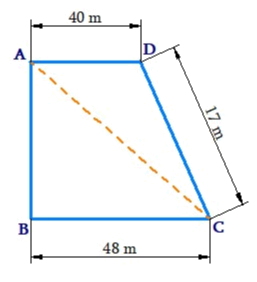### Solution

What is Known?

Length of the trapezium shaped field and side $$AB,\, DC,$$ and $$BC$$

What is unknown?

Area of the field and side $$AB.$$

Reasoning:

Visually area of given figure (trapezium) is sum of the area of two triangles.

Steps:

Length of the hence of a trapezium shaped field $$ABCD$$

\begin{align}&\!=\! AB + BC + CD + AD\\120\,{\rm{m }} &\!= \!AB \!+\! 48\,{\rm{m}} \!+\! 17\,{\rm{m }} \!+\! 40\,{\rm{m}}\\120\,{\rm{m}}& \!= \!AB \!+\! 105\,{\rm{m}}\\AB{\rm{ }} &= \!{\rm{ }}120\,{\rm{m }}\!-\!105\,{\rm{m}}\\ &=\! {\rm{ }}15\,{\rm{m}}\end{align}

Area of the field $$ABCD$$

\begin{align}&= \! \frac{1}{2} \! \times\! \! \begin{bmatrix}\text{Length of the}\\\text{ parallel side}\end{bmatrix}\!\! \times \! \begin{bmatrix}\text{Distance between } \\\text{two parallel sides}\end{bmatrix}\\& = \! \frac{1}{2} \! \times \! (AD \! + \! BC) \! \times \! AB\\&= \! \frac{1}{2} \! \times \! (40 \! + \! 48) \! \times \! 15\,{\rm{m}}\\& = \! \frac{1}{2} \! \times \! (88\,{\rm{m}}) \! \times \! 15\,{\rm{m}}\\& = \! 44\,{\rm{m}} \! \times \! 15\,{\rm{m}}\\&= \! 660\,{{\rm{m}}^2}\end{align}

Thus, Area of the field $$ABCD$$ is $$660\,{{\rm{m}}^2}$$.

## Chapter 11 Ex.11.2 Question 4

The diagonal of a quadrilateral shaped field is $$24\,\rm{ m}$$ and the perpendiculars dropped on it from the remaining opposite vertices are $$8\,\rm{ m}$$ and $$13\,\rm{ m.}$$ Find the area of the field.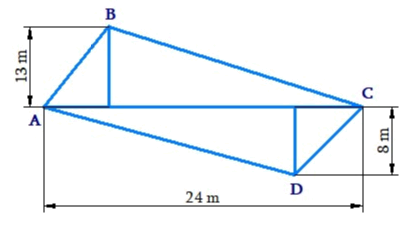### Solution

What is Known?

The shape of the field is quadrilateral, and length of the diagonal and length of the perpendicular drop is given.

What is unknown?

Area of the field $$ABCD.$$

Reasoning:

A general quadrilateral can be split into two triangles by drawing one of its diagrams .

Area for quadrilateral will be the sum of area of two triangles.

Steps:

Area of quadrilateral $$ABCD$$

\begin{align} &= \! \text{Area of }\Delta \,\text{ABC} \! + \! \text{Area of }\Delta \,\text{ADC} \\ & = \! \frac{1}{2} \! \times \! (AC \! \times \! BE) \! + \! \frac{1}{2} \! \times \! (AC \! \times \! FD) \\ & = \! \frac{1}{2} \! \times \! AC(BE \! + \! FD) \\ & = \! \frac{1}{2} \! \times \! 24\,\text{m}(13\,\text{m} \! +8 \! \,\text{m}) \\& = \! \frac{1}{2} \! \times \! 24\,\text{m} \! \times \! 21\,\text{m} \\ & = \! 252\,{{\text{m}}^{2}}\end{align}

Thus, area of the field is $$252\,{{\rm{m}}^2}$$

## Chapter 11 Ex.11.2 Question 5

The diagonals of a rhombus are $$7.5\,\rm{ cm}$$ and $$12\,\rm{ cm.}$$ Find its area.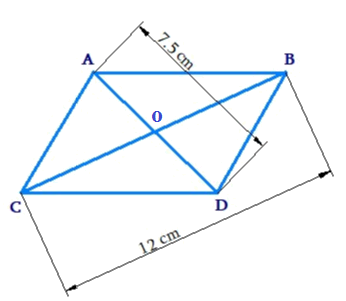### Solution

What is Known?

Diagonals of the rhombus are given.

What is unknown?

Area of rhombus.

Reasoning:

By using the method of splitting into triangles (triangulation method) we can find area of the rhombus visually diagonals $$AD$$ and $$BC$$ are perpendicular bisectors of each other hence area of rhombus $$ABCD$$ will be the sum of area of triangle $$ACB$$ and area of the triangle $$DBC.$$

Steps:

Area of Rhombus $$ABCD$$

\begin{align} &= {\text{Area of }}\Delta \,ABC + {\text{Area of}}\,\Delta \,DCB\\ &= \frac{1}{2} \times (CB \times AO) + \frac{1}{2} \times (CB \times OD)\\&= \frac{}{2}CB \times (AO + OD)\\ &= \frac{1}{2} \times CB \times AD\\& = \frac{1}{2} \times 12\,{\rm{m}} \times 7.5\,{\rm{m}}\\ &= 45.0\,{{\rm{m}}^2}\end{align}

Thus, area of the rhombus is $$45.0\,{{\rm{m}}^2}$$

## Chapter 11 Ex.11.2 Question 6

Find the area of a rhombus whose side is $$5\,\rm{ cm}$$ and whose altitude is $$4.8\,\rm{ cm.}$$ If one of its diagonals is $$8 \,\rm{cm}$$ long, find the length of the other diagonal.

### Solution

What is Known?

One of the diagonal, side and attitude of the rhombus.

What is unknown?

Area of the rhombus and length of the diagonal.

Reasoning:

Rhombus is a special case of parallelogram and the area of parallelogram is product of its base and height.

Steps: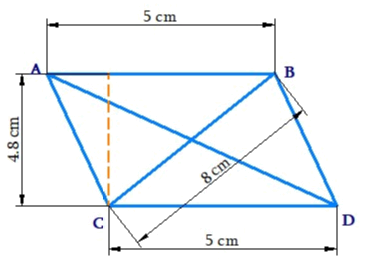Let the length of the other diagonal of rhombus is $$x.$$

Area of the rhombus $$ABCD$$

\begin{align} &= {\text{Base}} \times {\text{Length}}\\& = 5\,{\rm{cm}} \times 4.8 \, \rm{cm}\\ &= 24.0\,{{\rm{cm}}^2}\end{align}

Also,

Area of rhombus $$=\frac{1}{2}\times$$ Product of its diagonals

\begin{align} 24\,\text{cm}^{2} &=\frac{1}{2}(AD\times CB) \\ 24\,\text{cm}^{2}&=\frac{1}{2}(x\times 8\,\text{cm}) \\ x\times 4\,\text{cm}&=24\,\text{c}{{\text{m}}^{2}} \\ x&=6\,\text{cm}\end{align}

Thus, area of the rhombus is $$24.0\,{{\rm{m}}^2}$$ and length of the diagonals is $$6\,{\rm{cm}}$$ .

## Chapter 11 Ex.11.2 Question 7

The floor of a building consists of $$3000$$ tiles which are rhombus shaped and each of its diagonals is $$45 \,\rm{cm}$$ and $$30\,\rm{ cm}$$ in length. Find the total cost of polishing the floor, if the cost per $$\rm{m}^2$$ is $$4.$$

### Solution

What is Known?

The tiles used are rhombus shaped. Dimensions of single tile used and cost per $$\,{m^2}$$ of polishing the floor.

What is unknown?

Total cost of polishing the floor.

Reasoning:

By using the method of splitting into triangles (triangulation method) we can find area of the rhombus visually diagonals $$AD$$ and $$BC$$ are perpendicular bisectors of each other hence area of rhombus $$ABCD$$ will be the sum of area of triangle $$ACB$$ and area of the triangle $$DBC.$$

Steps: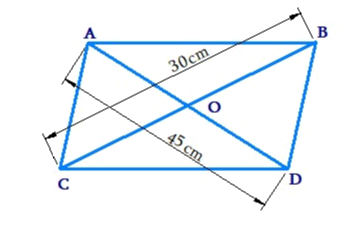Area of rhombus

\begin{align}& = \! {\text{Area of }}\Delta ABC \! + \! {\text{Area of }}\Delta DCB\\& = \! \frac{1}{2} \! \times \! (BC \! \times \! AO) \! + \! \frac{1}{2} \! \times \! (BC \! \times \! OD)\\& = \! \frac{1}{2} \! \times \! BC \! \times \! (AO \! + \! OD)\\&= \! \frac{1}{2} \! \times \! BC \! \times \! AD\\&= \! \frac{1}{2} \! \times \! 45\,{\rm{cm}} \! \times \! 30\,{\rm{cm}}\\& = \! 675\,{\rm{c}}{{\rm{m}}^2}\\\end{align}

Area of rhombus $$ABCD$$

\begin{align} &= \! \text{Area of }\Delta ABC \! + \! {\text{Area of }}\Delta DCB\\ &= \! \frac{1}{2} \! \times \! (BC \! \times \! AO) \! + \! \frac{1}{2} \! \times \! (BC \! \times \! OD)\\&= \! \frac{1}{2} \! \times \! BC \! \times \! (AO \! + \! OD)\\& = \! \frac{1}{2} \! \times \! BC \! \times \! AD\\&= \! \frac{1}{2} \! \times \! 45\,{\rm{cm}} \! \times \! 30\,{\rm{cm}}\\& = \! 675\,{\rm{c}}{{\rm{m}}^2}\end{align}

Area of each tile $$= 675\,\rm{m^2}$$

Area covered by $$3000$$ tiles

\begin{align}& = (675 \times 3000){\rm{c}}{{\rm{m}}^2}\\& = 2025000\,{\rm{c}}{{\rm{m}}^2}\\ &= 202.5\,{{\rm{m}}^2}\end{align}

The cost of polishing is $$\rm{Rs.}\, 4$$ per $${m^2}$$.

$$\therefore$$ Cost of polishing for $$202.5\,\rm{m^2}$$area $$= \rm{Rs.}(4 \times 202.5) = \rm{Rs.}810.0$$

Thus, the cost of polishing the floor is $$\rm{Rs }\,810.$$

## Chapter 11 Ex.11.2 Question 8

Mohan wants to buy a trapezium shaped field. Its side along the river is parallel to and twice the side along the road. If the area of this field is $$10500\;\rm{m^2}$$and the perpendicular distance between the two parallel sides is $$100\,\rm{m}$$, find the length of the side along the river.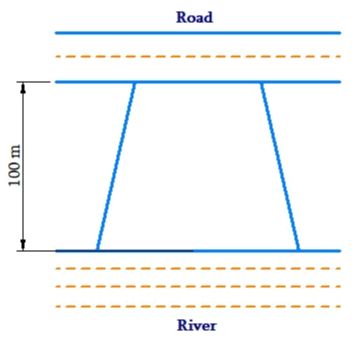### Solution

What is Known?

The field is trapezium shaped. The area of this field and perpendicular distance between the two parallel sides are known.

What is unknown?

Length of the field side along the river.

Reasoning:

Since area of the trapezium is known. So is easy to find another parallel side by using given information.

Steps:

Let the length of the field along the road be $$l$$ meter

Hence the length of the of the field along the river will be $$2l$$ meter

Area of the trapezium=$$\frac{1}{2}\times$$ (Sum of parallel side)$$\times$$ (Distance between the parallel sides)
\begin{align}10500\,\,{{\text{m}}^{2}}&=\frac{1}{2}\times (l+2l)\times 100\,\,\text{m} \\10500\,\,{{\text{m}}^{2}}&=\frac{1}{2}\times 3l\times 100\,\,\text{m} \\10500\,{{\text{m}}^{2}}&=3l\times 50 \,\rm{m} \\150\times l&=10500\,\text{m} \\l&=70\,\,\text{m}\end{align}

Thus, length of the field along the river $$= 2 \times l = 2 \times 70\,\,{\rm{m}} = 140\,\,{\rm{m}}$$.

## Chapter 11 Ex.11.2 Question 9

Top surface of a raised platform is in the shape of a regular octagon as shown in the figure. Find the area of the octagonal surface.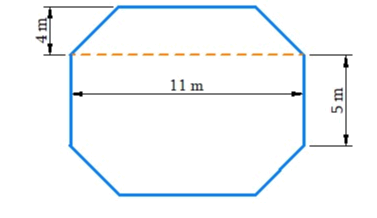### Solution

What is Known?

Top surface of a raised platform is octagonal shaped.

What is unknown?

Area of the octagonal surface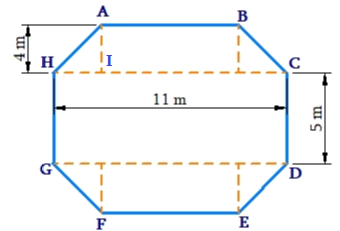Reasoning:

Visually, the area of the octagonal surface will be the sum of the area of two trapezium and area of rectangular.

Steps:

Area of octagon $$ABCDEFGH =$$ Area of trapezium $$ABCH\, +$$ Area of rectangular $$HCDG \;+$$ Area of trapezium $$EFGD$$

Side of the regular octagon $$= 5\,\rm{cm}$$

Area of trapezium $$ABCH\, =\,$$ Area of trapezium $$EFGD$$

Area of trapezium $$ABCH$$

\begin{align} &= \frac{1}{2} \times (AB + CH) \times AI\\&= \frac{1}{2} \times (5\,\rm{m} + 11\,\rm{m}) \times 4\,\rm{m}\\&= \frac{1}{2} \times 16\,\rm{m} \times 4\,\rm{m}\\&= 32\,\rm{m^2}\end{align}

$$\therefore$$ Area of trapezium $$ABCH\,=\,$$ Area of trapezium $$EFGD= 32 \rm{m^2}$$

Area of rectangle $$HCDG$$

\begin{align} &= HC \times CD \\&= 11{\rm{m}} \times 5{\rm{m}}\\& = 55{{\rm{m}}^2}\end{align}

Area of $$ABCDEFGH$$ $$=$$ Area of trapezium $$ABCH$$ $$+$$ Area of rectangle $$HCDG$$ $$+$$ Area of trapezium $$EFGD$$

\begin{align}& = 32\,{{\rm{m}}^2} + 55\,{{\rm{m}}^2} + 32\,{{\rm{m}}^2}\\& = 119\,\rm{m^2}\end{align}

Thus, the area of the octagonal surface is $$119{{\rm{m}}^2}$$

## Chapter 11 Ex.11.2 Question 10

There is a pentagonal shaped park as shown in the figure. For finding its area Jyoti and Kavita divided it in two different ways. Find the area of this park using both ways. Can you suggest some other way of finding its area?

### Solution

What is Known?

The park is pentagonal shape and dimension of the park is given.

What is unknown?

Area of the park

Reasoning:

Visually the pentagon is divided into two equal trapeziums or into one triangle and one square. So, the area of the pentagon will be sum of the area of triangle and area of the square.

Steps: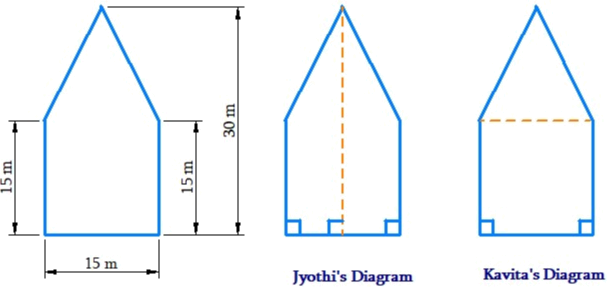(i) Jyoti’s way of triangle area is as follows

Area of pentagon $$ABCDE\,=$$ Area of trapezium $$ABCD\,+$$ Area of trapezium $$AEDF$$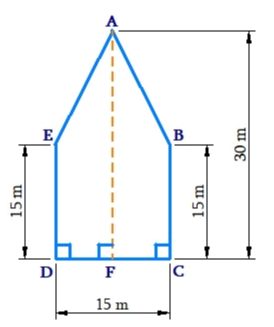\begin{align}& = \begin{bmatrix} \frac{1}{2} \times (AF + BC) \times FC +\\\frac{1}{2} \times (AF \times BC) \times DF\end{bmatrix}\\& =\begin{bmatrix} \frac{1}{2} \times (13\,{\rm{m}} + 15\,{\rm{m}}) \times \frac{{15}}{2}\,{\rm{m}} +\\ \frac{1}{2} \times (30 + 15{\rm{m}}) \times \frac{{15}}{2}{\rm{m}}\end{bmatrix}\\& = 2 \times \frac{1}{2}(30\,{\rm{m}} + 15\,{\rm{m}}) \times \frac{{15}}{2}\\& = 45\,{\rm{m}} \times 7.5\,{\rm{m}}\\& = 337.5\,{{\rm{m}}^2}\end{align}

Thus, area of the pentagonal shaped park according to Jyoti’s way is $$337.5\,\rm{m^2}$$

(ii) Kavitha’s way of finding area is as follows.

Area of pentagon $$ABCDE\;=$$ Area of triangle $$ABE\; +$$ Area of square $$EBDC$$

\begin{align}& = \! \frac{1}{2} \! \times \! BE \! \times \! (AF \! - \! OF) \! + \! FC \! \times \! BC\\ &= \! \frac{1}{2} \! \times \! 15 \! \times \! (30 \! - \! 15) \! + \! (15 \! \times \! 15\,\,{\rm{m}})\\ &= \! \left( {\frac{1}{2} \! \times \! 15 \! \times \! 15} \right){{\rm{m}}^2} \! + \! 225\,\,{{\rm{m}}^2}\\ &= \! 112.5\,\,{{\rm{m}}^2} \! + \! 225\,\,{{\rm{m}}^2}\\& = \! 337.5\,\,{{\rm{m}}^2}\end{align}

Thus, the area of the pentagonal shaped park according to Kavitha’s way is $$337.5 \,\rm{m^2}$$

Another way of finding its area is follows.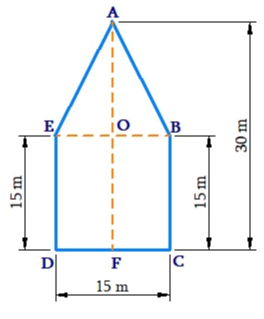Area of pentagon $$ABCDE \;=$$Area of rectangle $$GFCD – 2$$(Area of $$\Delta AGE$$)

\begin{align} &= \! 30\,{\rm{m}} \! \times \! 15\,\,{\rm{m}} \! - \! 2 \! \times \! \begin{bmatrix} {\frac{1}{2} \! \times \! 7.5 \! \times \! 1.5} \end{bmatrix}\\& = \! 450\,{{\rm{m}}^2} \! + \! 112.5\,\,{{\rm{m}}^2}\\& = \! 337.5\,{\rm{c}}{{\rm{m}}^2}\end{align}

## Chapter 11 Ex.11.2 Question 11

Diagram of the adjacent picture frame has outer dimensions $$= 24\,\rm{ cm} \times 28\,\rm{cm}$$ and inner dimensions $$16 \,\rm{cm} × 20\,\rm{ cm.}$$ Find the area of each section of the frame, if the width of each section is same.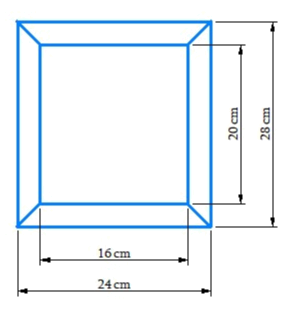### Solution

What is Known?

Inner and outer dimensions of the frame.

What is unknown?

Area of each section of the frame

Reasoning:

Visually, there are four trapezium and one rectangle in the given figure.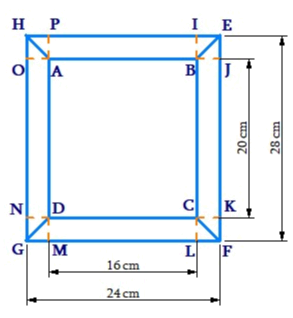Steps:

Given that the width of each section is same, therefore.

$$\left[\begin{array} =IB = BJ=CK=CL=\\DM=DN=AP=AO\end{array}\right]$$

\begin{align}IL &= IB + BC + CL\\28 &= IB + 20\,{\rm{cm}} + CL\\28\,{\rm{cm}} - 20\,{\rm{cm}}& = IB + CL\\8\,\rm{cm} &= IB + CL\\IB&= CL\\2IB &= 8\,{\rm{cm}}\\IB &= 4\,{\rm{m}}\end{align}

Hence

$$\left[\begin{array} =IB = BJ=CK=CL=\\DN=AP=AO=4\,\rm{cm}\end{array}\right]$$

Area of section $$ABEH=$$ Area of section $$CDFG$$

\begin{align} &= \frac{1}{2} \times (AB + HE) + IB\\ &= \frac{1}{2}(16 + 24) \times 4\,{\rm{cm}}\\ &= 80\,{{\rm{m}}^2}\end{align}

Area of section $$BEFC=$$ Area of section $$ADGH$$

\begin{align} &= \frac{1}{2} \times (BC + EF) + BJ\\& = \frac{1}{2} \times (20 + 28)\,{\rm{cm}} \times 4\,{\rm{cm}}\\ &= 96\,{{\rm{m}}^2}\end{align}

Area of section $$ABCD$$

\begin{align}&= BC \times DC\\ &= 20\,{\rm{cm}} \times 16\,{\rm{cm}}\\ &= 320\,{{\rm{m}}^2}\end{align}

Instant doubt clearing with Cuemath Advanced Math Program